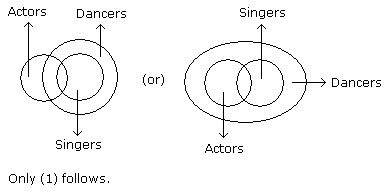# Verbal Reasoning - Syllogism - Discussion

Discussion Forum : Syllogism - Syllogism 1 (Q.No. 1)
Directions to Solve

In each of the following questions two statements are given and these statements are followed by two conclusions numbered (1) and (2). You have to take the given two statements to be true even if they seem to be at variance from commonly known facts. Read the conclusions and then decide which of the given conclusions logically follows from the two given statements, disregarding commonly known facts.

• (A) If only (1) conclusion follows
• (B) If only (2) conclusion follows
• (C) If either (1) or (2) follows
• (D) If neither (1) nor (2) follows and
• (E) If both (1) and (2) follow.

1.

Statements: Some actors are singers. All the singers are dancers.

Conclusions:

1. Some actors are dancers.
2. No singer is actor.

Only (1) conclusion follows
Only (2) conclusion follows
Either (1) or (2) follows
Neither (1) nor (2) follows
Both (1) and (2) follow
Explanation:Discussion:
71 comments Page 1 of 8.

Mosfar Ali said:   3 weeks ago
Agree, Option A is correct.
(2)

Gautam said:   1 month ago
Yes, @Waheed.

It's correct because there is no cross between actor, dancer or singer.
(1)

Ladshika Mohan said:   3 years ago
Here, 2nd diagram is wrong one and instead of that, the three circles become intersect and the inside circle mentioned singers next circle mentioned dancers and outside circle mentioned actors, this should be right.
(3)

WAHEED said:   5 years ago
How first conclusion follows? I think either is the right answer.
(8)

Tusar said:   5 years ago
@All.

Always solve from the conclusion. If both the statement have a common and all is attached with that common word. Then conclusion true. Ex here common is a singer. And singer Se pehele all he. So directly some actors are dancers. Next, for conclusion 2 we can't draw -ve conclusion from positive statements. So false.
(3)

Huraira said:   6 years ago
@SUMAN

you repeated
B+A = Y.
B +B = no conclusion.
B+Z = Y.
B+Y= no conclusion.

B+A = Z.
B +B = Y.
B+Z = no conclusion.
B+Y= no conclusion.

Which combination is now correct?
(1)

Suman said:   6 years ago
Let A- all (eg- all trees are dog.)
B- all not (eg- all trees are not dog.)
Z- some (eg- some trees are dog.)
Y- some not(eg- some trees are not dog.)

A can be converted to Z(eg- All trees are dog. conversion- some trees are dogs. (or) some dogs are tree)
B can be converted to B or Y((eg- All trees are not dog. conversion- all dogs are not tree. (or) All trees are not dog))
Z can be converted to z(eg- some trees are dogs.conversion- some dogs are trees.)
Y has no conclusion.

Combination :
A+A = Z.
A +B = Z.
A+Z = no conclusion.
A+Y= no conclusion.

B+A = Y.
B +B = no conclusion.
B+Z = Y.
B+Y= no conclusion.

B+A = Z.
B +B = Y.
B+Z = no conclusion.
B+Y= no conclusion.

Y + (A, B, Z)= no conclusion.
(2)

Kamal said:   6 years ago
Hi,

Can anyone explain it in short? Because I couldn't get the "some are not " model.

Statements:
All squares are circles.
All circles are units.
No circle is a meter.

Conclusions:

I. Some units which are not circles can be meters.
II. Some squares being meters is a possibility.

Anyone, please explain how to solve this using formula table.

Ravi kumar said:   6 years ago
2nd Venn diagram shows that all actors are dancers. Then, why option A is correct?
(1)

Roshan said:   7 years ago
In second diagram. All actors are dancer comes out. And in first some actors are dancers. If the statement is not satisfying both the diagrams, then how we can say one is correct?